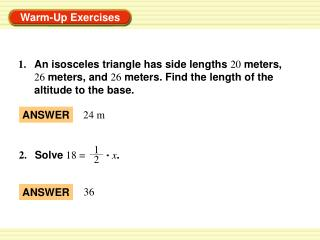Download Presentation1. An isosceles triangle has side lengths 20 meters,1. An isosceles triangle has side lengths 20 meters, - PowerPoint PPT Presentation

Download Presentation1. An isosceles triangle has side lengths 20 meters,
An Image/Link below is provided (as is) to download presentation

Download Policy: Content on the Website is provided to you AS IS for your information and personal use and may not be sold / licensed / shared on other websites without getting consent from its author. While downloading, if for some reason you are not able to download a presentation, the publisher may have deleted the file from their server.

- - - - - - - - - - - - - - - - - - - - - - - - - - - E N D - - - - - - - - - - - - - - - - - - - - - - - - - - -
Presentation Transcript

1. ANSWER 24 m 1 2. Solve 18 = x. 2 36 ANSWER 1. An isosceles triangle has side lengths 20 meters, 26 meters, and 26 meters. Find the length of the altitude to the base.

2. ANSWER 13.47 10 4. Evaluate . tan 88º ANSWER 0.3492 3. Evaluate (4 cos 10º)(10 sin 20º).

3. In the diagram, ABCDEis a regular pentagon inscribed in F. Find each angle measure. 360° 5 a. m AFB AFB is a central angle,som AFB = , or 72°. Find angle measures in a regular polygon EXAMPLE 1 SOLUTION

4. In the diagram, ABCDEis a regular pentagon inscribed in F. Find each angle measure. b. m AFG FG is an apothem, which makes it an altitude ofisosceles ∆AFB. So, FGbisectsAFB andm AFG = m AFB = 36°. 1 2 Find angle measures in a regular polygon EXAMPLE 1 SOLUTION

5. In the diagram, ABCDEis a regular pentagon inscribed in F. Find each angle measure. c. m GAF The sum of the measures of right ∆GAF is 180°. So, 90° + 36° + m GAF = 180°, andm GAF = 54°. Find angle measures in a regular polygon EXAMPLE 1 SOLUTION

6. In the diagram, WXYZis a square inscribed in P. 1. Identify the center, a radius, an apothem, and a central angle of the polygon. ANSWER P, PY or XP, PQ, XPY. for Example 1 GUIDED PRACTICE

7. 2. Find m XPY, m XPQ, andm PXQ. ANSWER 90°, 45°, 45° for Example 1 GUIDED PRACTICE

8. You are decorating the top of a table by covering it with small ceramic tiles. The table top is a regular octagon with 15inch sides and a radius of about 19.6inches. What is the area you are covering? Find the area of a regular polygon EXAMPLE 2 DECORATING SOLUTION STEP 1 Find the perimeter Pof the table top. An octagon has 8 sides, so P = 8(15) = 120inches.

9. Find the apothem a. The apothem is height RSof ∆PQR. Because ∆PQRis isosceles, altitude RSbisects QP. 1 1 So,QS = (QP) = (15) = 7.5 inches. 2 2 √ a = RS ≈ √19.62 – 7.52 = 327.91 ≈ 18.108 Find the area of a regular polygon EXAMPLE 2 STEP 2 To find RS, use the Pythagorean Theorem for ∆ RQS.

10. 1 A = aP 2 1 ≈ (18.108)(120) 2 ANSWER So, the area you are covering with tiles is about 1086.5square inches. Find the area of a regular polygon EXAMPLE 2 STEP 3 Find the area Aof the table top. Formula for area of regular polygon Substitute. ≈ 1086.5 Simplify.

11. A regular nonagon is inscribed in a circle with radius 4 units. Find the perimeter and area of the nonagon. SOLUTION 360° The measure of central JLKis , or 40°. Apothem LMbisects the central angle, so m KLMis 20°. To find the lengths of the legs, use trigonometric ratios for right ∆KLM. 9 Find the perimeter and area of a regular polygon EXAMPLE 3

12. LM MK sin 20° = cos 20° = LK LK MK LM cos 20° = sin 20° = 4 4 4 sin 20° = MK 4 cos 20° = LM The regular nonagon has side length s = 2MK = 2(4 sin 20°) = 8  sin 20° and apothem a = LM = 4  cos20°. Find the perimeter and area of a regular polygon EXAMPLE 3

13. ANSWER So, the perimeter is P = 9s = 9(8 sin 20°) = 72 sin 20° ≈ 24.6 units, and the area is A = aP = (4 cos 20°)(72 sin20°)≈46.3 square units. 1 1 2 2 Find the perimeter and area of a regular polygon EXAMPLE 3

14. 3. ANSWER about 46.6 units, about 151.5 units2 for Examples 2 and 3 GUIDED PRACTICE Find the perimeter and the area of the regular polygon.

15. 4. ANSWER 70 units, about 377.0 units2 for Examples 2 and 3 GUIDED PRACTICE Find the perimeter and the area of the regular polygon.

16. 5. 30 3  52.0units, about 129.9 units2 ANSWER for Examples 2 and 3 GUIDED PRACTICE Find the perimeter and the area of the regular polygon.

17. ANSWER Exercise 5 for Examples 2 and 3 GUIDED PRACTICE 6. Which of Exercises 3–5 above can be solved using special right triangles?

18. ANSWER 15° 2. ANSWER 110 cm2 Daily Homework Quiz 1. Find the measure of the central angle of a regular polygon with 24 sides. Find the area of each regular polygon.

19. 3. ANSWER 374.1 cm2 Daily Homework Quiz

20. 4. ANSWER 99.4 in. ; 745.6 in.2 Daily Homework Quiz Find the perimeter and area of each regular polygon.

21. 5. 22.6 m; 32 m2 ANSWER Daily Homework Quiz